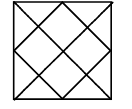How many triangles are there in figure given below?

# How many triangles are there in figure given below?1. A

20

2. B

27

3. C

18

4. D

29

Fill Out the Form for Expert Academic Guidance!l

+91

Live ClassesBooksTest SeriesSelf Learning

Verify OTP Code (required)

### Solution:

No. of triangles

1 Unit:- $1,\text{\hspace{0.17em}}2,\text{\hspace{0.17em}}3,\text{\hspace{0.17em}}5,\text{\hspace{0.17em}}8,\text{\hspace{0.17em}}11,\text{\hspace{0.17em}}12,\text{\hspace{0.17em}}10\to 8$

2 Unit:- $\left(1,\text{\hspace{0.17em}}2\right)\text{\hspace{0.17em}\hspace{0.17em}}\left(3,\text{\hspace{0.17em}}5\right)\text{\hspace{0.17em}\hspace{0.17em}}\left(8,\text{\hspace{0.17em}}11\right)\text{\hspace{0.17em}\hspace{0.17em}}\left(12,10\right)\to 4$

3 Unit:- $\left(1,\text{\hspace{0.17em}}6,\text{\hspace{0.17em}}8\right)\text{\hspace{0.17em}\hspace{0.17em}}\left(9,\text{\hspace{0.17em}}11,\text{\hspace{0.17em}}12\right)\text{\hspace{0.17em}\hspace{0.17em}}\left(7,\text{\hspace{0.17em}}5,\text{\hspace{0.17em}}10\right)\text{\hspace{0.17em}\hspace{0.17em}}\left(2,\text{\hspace{0.17em}}3,\text{\hspace{0.17em}}4\right)\to 4$

6 Unit:- $\left(1,\text{\hspace{0.17em}}6,\text{\hspace{0.17em}}9,\text{\hspace{0.17em}}12,\text{\hspace{0.17em}}11,\text{\hspace{0.17em}}8\right)\text{\hspace{0.17em}\hspace{0.17em}}\left(2,\text{\hspace{0.17em}}4,\text{\hspace{0.17em}}3,\text{\hspace{0.17em}}5,\text{\hspace{0.17em}}7,\text{​\hspace{0.17em}}10\right)\to 2$

Total = 18+91

Live ClassesBooksTest SeriesSelf Learning

Verify OTP Code (required)